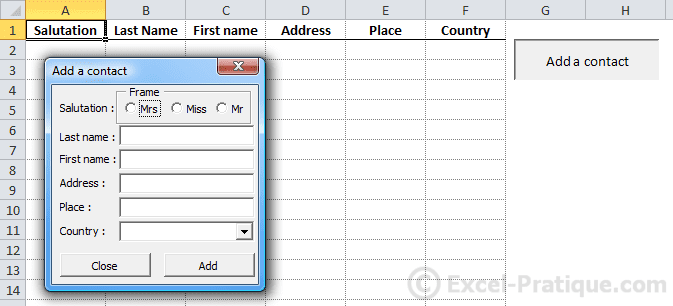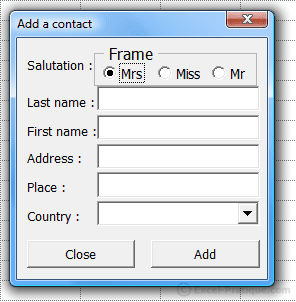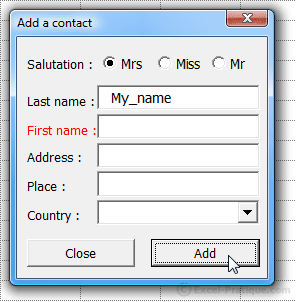# VBA Course: Controls (exercise)

Now let's try a little exercise to practice using controls ...

This is how we start the exercise:

Here is the downloadable Excel file: controls_exercise.xlsBy now it should be clear that the goal here is to fill the table using the form.

A few thinks to keep in mind:

• List the countries based on the list on the second worksheet
• Verify the contents of the controls before adding a new contact
• After inserting a value, reinitialize the values of the controls without closing the form

Take a moment to work through this exercise before looking at the solution ...

.
.
.
.
.
.
.
.
.
.
.
.
.
.
.
.
.
.
.
.
.
.
.
.
.
.
.
.
.
.

## This is one way that you could solve this problem

Our first action should be to increase the Zoom property of the UserForm to 120 to make it easier to use the form:We have already covered option button tests (in the first controls page), so we are using a simpler solution here.

"Mrs" is chosen by default (Value property: True), which means that we don't have to verify that a salutation has been chosen.

## The "Close" button

``````Private Sub CommandButton_Close_Click()
End Sub``````

## The contents of the drop-down list

``````Private Sub UserForm_Initialize() 'Loading the list when the UserForm is opened
For i = 1 To 252 'List of 252 countries froim the "Country" worksheet
Next
End Sub``````

## Verification of controls

One simple solution would be to display a dialog box if any of the controls is empty:

``````Private Sub CommandButton_Add_Click()
If TextBox_Last_Name.Value = "" Or TextBox_First_Name.Value = "" Or TextBox_Address.Value = "" Or TextBox_Place.Value = "" Or ComboBox_Country.Value = "" Then
MsgBox "Form incomplete"
Else
'Instructions for inserting a contact here ...
End If
End Sub``````

But to make things a bit more complicated, each control should be tested individually, and if any of them is empty, its Label text color should be changed to red:

``````Private Sub CommandButton_Add_Click()
'Setting Label text color to black
Label_Last_Name.ForeColor = RGB(0, 0, 0)
Label_First_Name.ForeColor = RGB(0, 0, 0)
Label_Address.ForeColor = RGB(0, 0, 0)
Label_Place.ForeColor = RGB(0, 0, 0)
Label_Country.ForeColor = RGB(0, 0, 0)

'Content controls
If TextBox_Last_Name.Value = "" Then 'IF no "name" provided ...
Label_Last_Name.ForeColor = RGB(255, 0, 0) 'Set Label "name" color to red
ElseIf TextBox_First_Name.Value = "" Then
Label_First_Name.ForeColor = RGB(255, 0, 0)
ElseIf TextBox_Address.Value = "" Then
Label_Address.ForeColor = RGB(255, 0, 0)
ElseIf TextBox_Place.Value = "" Then
Label_Place.ForeColor = RGB(255, 0, 0)
ElseIf ComboBox_Country.Value = "" Then
Label_Country.ForeColor = RGB(255, 0, 0)
Else
'Instructions for inserting a contact here ...
End If
End Sub``````## Inserting data

The following code should be inserted in the place indicated in the code above (see commentary):

``````Dim row_number As Integer, salutation As String

'Choice of Salutation
For Each salutation_button In Frame_Salutation.Controls
If salutation_button.Value Then
salutation = salutation_button.Caption 'Salutation chosen
End If
Next

'row_number = row number of the last non-empty cell in the column +1
row_number = Range("A65536").End(xlUp).Row + 1

'Inserting values into the worksheet
Cells(row_number, 1) = salutation
Cells(row_number, 2) = TextBox_Last_Name.Value
Cells(row_number, 3) = TextBox_First_Name.Value
Cells(row_number, 4) = TextBox_Address.Value
Cells(row_number, 5) = TextBox_Place.Value
Cells(row_number, 6) = ComboBox_Country.Value

'After insertion, the initial values are replaced
OptionButton1.Value = True
TextBox_Last_Name.Value = ""
TextBox_First_Name.Value = ""
TextBox_Place.Value = ""
ComboBox_Country.ListIndex = -1``````

## Overall view

That's all, and here you have the complete code for the exercise and the downloadable Excel file:

``````Private Sub CommandButton_Close_Click()
End Sub

Private Sub UserForm_Initialize() 'List of 252 countries on the "Country" worksheet
For i = 1 To 252
Next
End Sub

'Setting Label color to black
Label_Last_Name.ForeColor = RGB(0, 0, 0)
Label_First_Name.ForeColor = RGB(0, 0, 0)
Label_Address.ForeColor = RGB(0, 0, 0)
Label_Place.ForeColor = RGB(0, 0, 0)
Label_Country.ForeColor = RGB(0, 0, 0)

'Content controls
If TextBox_Last_Name.Value = "" Then 'If no "name" provided ...
Label_Last_Name.ForeColor = RGB(255, 0, 0) 'Set Label "name" color to red
ElseIf TextBox_First_Name.Value = "" Then
Label_First_Name.ForeColor = RGB(255, 0, 0)
ElseIf TextBox_Address.Value = "" Then
Label_Address.ForeColor = RGB(255, 0, 0)
ElseIf TextBox_Place.Value = "" Then
Label_Place.ForeColor = RGB(255, 0, 0)
ElseIf ComboBox_Country.Value = "" Then
Label_Country.ForeColor = RGB(255, 0, 0)
Else
'If the form is complete, the values will be inserted onto the worksheet
Dim row_number As Integer, salutation As String

'Choice of salutation
For Each salutation_button In Frame_Salutation.Controls
If salutation_button.Value Then
salutation = salutation_button.Caption
End If
Next

'row_number = row number of the last non-empty cell in column +1
row_number = Range("A65536").End(xlUp).Row + 1

'Inserting values onto the worksheet
Cells(row_number, 1) = salutation
Cells(row_number, 2) = TextBox_Last_Name.Value
Cells(row_number, 3) = TextBox_First_Name.Value
Cells(row_number, 4) = TextBox_Address.Value
Cells(row_number, 5) = TextBox_Place.Value
Cells(row_number, 6) = ComboBox_Country.Value

'After insert, we replace the initial values
OptionButton1.Value = True
TextBox_Last_Name.Value = ""
TextBox_First_Name.Value = ""